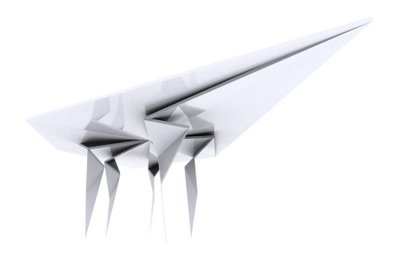## Wednesday, July 19, 2006

### Rh4_060719_SubDiv_Progress...

---------FRACTAL---------

The term fractal was coined in 1975 by Benoît Mandelbrot, from the Latin fractus, meaning "broken" or "fractured." In colloquial usage, a fractal is a shape that is recursively constructed or self-similar, that is, a shape that appears similar at all scales of magnification and is therefore often referred to as "infinitely complex." Mathematicians avoid giving the strict definition and prefer to call 'fractal a geometric object that usually.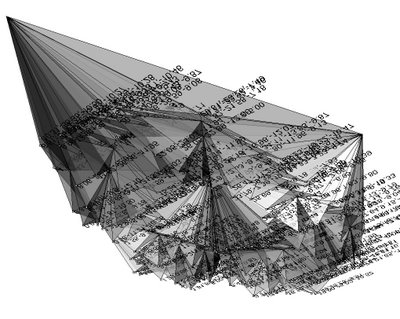GENERATING FRACTALS
Three common techniques for generating fractals are:

1. ITERATED FUNCTION SYSTEMS — These have a fixed geometric replacement rule. Cantor set, Sierpinski carpet, Sierpinski gasket, Peano curve, Koch snowflake, Harter-Heighway dragon curve, T-Square, Menger sponge, are some examples of such fractals.

2. ESCAPE-TIME FRACTALS — Fractals defined by a recurrence relation at each point in a space (such as the complex plane). Examples of this type are the Mandelbrot set, the Burning Ship fractal and the Lyapunov fractal.

3. RANDOM FRACTALS — Generated by stochastic rather than deterministic processes, for example, fractal landscapes, Lévy flight and the Brownian tree. The latter yields so-called mass- or dendritic fractals, for example, diffusion-limited aggregation or reaction-limited aggregation clusters.

CLASSIFICATION OF FRACTALS
Fractals can also be classified according to their self-similarity. There are three types of self-similarity found in fractals:

1. EXACT SELF-SIMILARITY — This is the strongest type of self-similarity; the fractal appears identical at different scales. Fractals defined by iterated function systems often display exact self-similarity.

2. QUASI SELF-SIMILARITY — This is a loose form of self-similarity; the fractal appears approximately (but not exactly) identical at different scales. Quasi-self-similar fractals contain small copies of the entire fractal in distorted and degenerate forms. Fractals defined by recurrence relations are usually quasi-self-similar but not exactly self-similar.

3. STATISTICAL SELF-SIMILARITY — This is the weakest type of self-similarity; the fractal has numerical or statistical measures which are preserved across scales. Most reasonable definitions of "fractal" trivially imply some form of statistical self-similarity. (Fractal dimension itself is a numerical measure which is preserved across scales.) Random fractals are examples of fractals which are statistically self-similar, but neither exactly nor quasi-self-similar.

'------------------------------------------------------------
"diamonds are for ever..." as should be the such type of structures...
here, the speculation is to carry the load down at the gravity pt for structural stability...
the code is starting from a closed polyline, subdividing the created project surface into triangles (or arrays of 3pts), locating areas centroides, changing their Z value and looping (fractal subdivision)...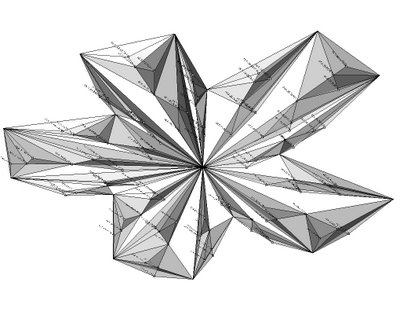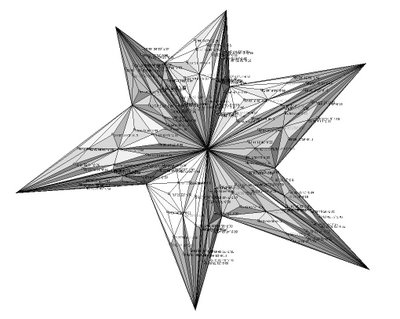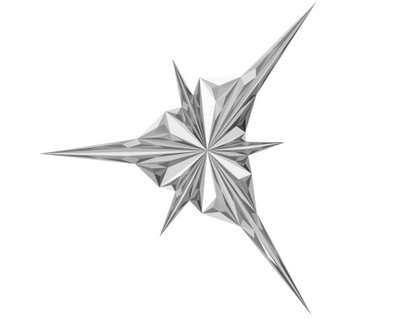## Thursday, July 13, 2006

### Rh4_060713_SubDiv

---------SUBDIVISION SURFACES---------

In computer graphics, SUBDIVISION SURFACES are used to create smooth surfaces out of arbitrary meshes. Subdivision surfaces are defined as the limit of an infinite refinement process. They were introduced simultaneously by Edwin Catmull and Jim Clark, and by Daniel Doo and Malcom Sabin in 1978. Little progress was made until 1995, when Ulrich Reif solved subdivision surfaces behaviour near extraordinary vertices.

The fundamental concept is REFINEMENT. By repeatedly refining an initial polygonal mesh, a sequence of meshes is generated that converges to a resulting subdivision surface. Each new subdivision step generates a new mesh that has more polygonal elements and is smoother.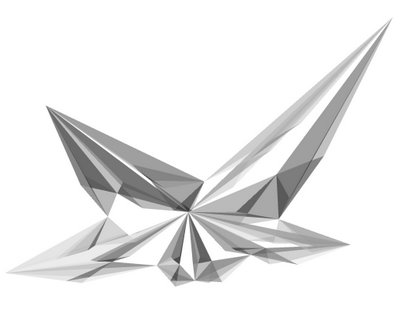Here is a first code on a research for a set of self similar furnitures based on fractal diamond subdivision...
This routine is similar to a very interesting "Cracking algorythm" developped by Aranda/Larsch (i.e. Terraswarm link).

Sub SubDivDiamond()
' ------------------------------------------------------
' getPts
Dim arrPts00: arrPts00 = Rhino.GetPoints(vbTrue)
Dim dblDeletaHeight: dblDeletaHeight = 0
' ------------------------------------------------------
' LOOP_00
' set firstCentroide
Dim arrMP00: arrMP00 = Rhino.CurveAreaCentroid(strPoly00)
' set firstCentroide_Height
Dim arrMP00_deltaHeight: arrMP00_deltaHeight = Array( arrMP00(0)(0), arrMP00(0)(1), arrMP00(0)(2) + dblDeletaHeight )
arrMP00(0) = arrMP00_deltaHeight
' set arrays of 3pts each
Dim i, arrPtsTank00()
For i = 1 To UBound (arrPts00)
ReDim Preserve arrPtsTank00(i-1)
arrPtsTank00(i-1) = Array( arrMP00(0), arrPts00(i-1), arrPts00(i) )
Next
' ------------------------------------------------------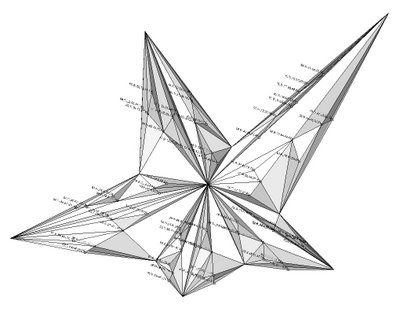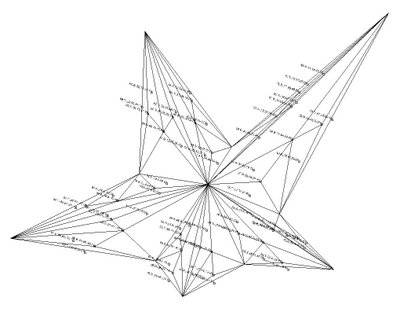' LOOPS
Dim n1, n2, n3
Dim arrPtsTank01(), arrPtsTank02(), arrPtsTank03()

For n1 = 0 To UBound(arrPtsTank00)
ReDim Preserve arrPtsTank01(n1)
arrPtsTank01(n1) = Subdiv (arrPtsTank00(n1))

For n2 = 0 To UBound(arrPtsTank01(n1))
ReDim Preserve arrPtsTank02(n2)
arrPtsTank02(n2) = Subdiv (arrPtsTank01(n1)(n2))

For n3 = 0 To UBound(arrPtsTank02(n2))
ReDim Preserve arrPtsTank03(n3)
arrPtsTank03(n3) = Subdiv (arrPtsTank02(n2)(n3))
Next

Next

Next

' ------------------------------------------------------
End Sub
SubDivDiamond
' ------------------------------------------------------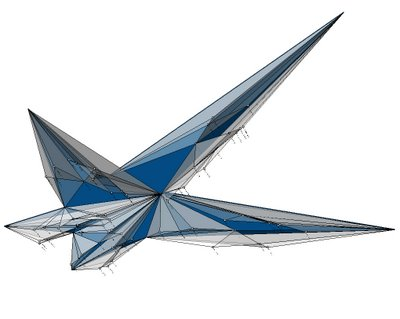' ------------------------------------------------------
Function Subdiv(arrPtsInput)
Dim j
Dim arrPtsTank()
Dim dblDeletaHeight2: dblDeletaHeight2 = -15

Dim strPoly: strPoly = Rhino.AddPolyline (Array (arrPtsInput(0),arrPtsInput(1),arrPtsInput(2), arrPtsInput(0)) )
Dim arrMP: arrMP = Rhino.CurveAreaCentroid(strPoly)
' Centroide_Height
Dim arrMP_deltaHeight: arrMP_deltaHeight = Array( arrMP(0)(0), arrMP(0)(1), arrMP(0)(2) + dblDeletaHeight2 )
arrMP(0) = arrMP_deltaHeight
' Centroide_Annotation
Dim arrPtCentroid: arrPtCentroid = Rhino.AddPoint (arrMP(0))

For j = 1 To UBound (arrPtsInput)
' set Arrays of 3pts each
ReDim Preserve arrPtsTank(j-1)
arrPtsTank(j-1) = Array( arrMP(0), arrPtsInput(j-1), arrPtsInput(j) )